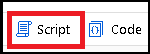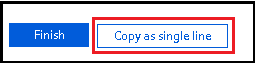# 数据流脚本 (DFS)## 用例

DFS 由用户界面自动生成。 可以单击“脚本”按钮来查看并自定义该脚本。 还可以在 ADF UI 外部生成脚本，然后将其传递到 PowerShell cmdlet。 调试复杂数据流时，你可能会发现扫描脚本代码隐藏比扫描流的 UI 图形表示形式更容易。

• 以编程方式生成许多非常相似的数据流，即“标记”数据流。
• 在 UI 中难以管理或者会导致验证问题的复杂表达式。
• 调试和更好地了解执行过程中返回的各种错误。## 如何添加转换

``````source(output(
movieId as string,
title as string,
genres as string
),
validateSchema: false) ~> source1
validateSchema: false) ~> sink1
``````

``````derive(upperCaseTitle = upper(title)) ~> deriveTransformationName
``````

``````source(output(
movieId as string,
title as string,
genres as string
),
validateSchema: false) ~> source1
derive(upperCaseTitle = upper(title)) ~> deriveTransformationName
validateSchema: false) ~> sink1
``````

``````source(output(
movieId as string,
title as string,
genres as string
),
validateSchema: false) ~> source1
source1 derive(upperCaseTitle = upper(title)) ~> deriveTransformationName
validateSchema: false) ~> sink1
``````

``````source(output(
movieId as string,
title as string,
genres as string
),
validateSchema: false) ~> source1
source1 derive(upperCaseTitle = upper(title)) ~> deriveTransformationName
validateSchema: false) ~> sink1
``````

## DFS 基础知识

DFS 由一系列连接的转换组成，其中包括源、接收器，以及各种其他转换（可添加新列、筛选数据、联接数据以及执行其他操作）。 通常情况下，脚本以一个或多个源开始，后跟许多转换，以一个或多个接收器结束。

``````source(
source properties
) ~> source_name
``````

``````source(output(
movieId as string,
title as string,
genres as string
),
validateSchema: false) ~> source1
``````

``````name_of_incoming_stream transformation_type(
properties
) ~> new_stream_name
``````

``````source1 derive(
title = upper(title)
) ~> derive1
``````

``````derive1 sink(allowSchemaDrift: true,
validateSchema: false) ~> sink1
``````

## 脚本代码片段

### 聚合汇总统计信息

``````aggregate(each(match(true()), \$\$+'_NotNull' = countIf(!isNull(\$\$)), \$\$ + '_Null' = countIf(isNull(\$\$))),
each(match(type=='double'||type=='integer'||type=='short'||type=='decimal'), \$\$+'_stddev' = round(stddev(\$\$),2), \$\$ + '_min' = min (\$\$), \$\$ + '_max' = max(\$\$), \$\$ + '_average' = round(avg(\$\$),2), \$\$ + '_variance' = round(variance(\$\$),2)),
each(match(type=='string'), \$\$+'_maxLength' = max(length(\$\$)))) ~> SummaryStats
``````

``````aggregate(groupBy(title),
countunique = count()) ~> ValueDistAgg
ValueDistAgg aggregate(numofunique = countIf(countunique==1),
numofdistinct = countDistinct(title)) ~> UniqDist
``````

### 包含聚合中的所有列

``````aggregate(groupBy(movie),
each(match(name!='movie'), \$\$ = first(\$\$))) ~> DistinctRows
``````

### 创建行哈希指纹

``````derive(DWhash = sha1(Name,ProductNumber,Color)) ~> DWHash
``````

``````derive(DWhash = sha1(columns())) ~> DWHash
``````

### String_agg 等效项

``````source1 aggregate(groupBy(year),
string_agg = collect(title)) ~> Aggregate1
Aggregate1 derive(string_agg = toString(string_agg)) ~> StringAgg
``````

### 更新、更新插入、插入、删除的次数

``````aggregate(updates = countIf(isUpdate(), 1),
inserts = countIf(isInsert(), 1),
upserts = countIf(isUpsert(), 1),
deletes = countIf(isDelete(),1)) ~> RowCount
``````

### 使用所有列的非重复行

``````aggregate(groupBy(mycols = sha2(256,columns())),
each(match(true()), \$\$ = first(\$\$))) ~> DistinctRows
``````

### 检查所有列中是否存在 NULL 值

``````split(contains(array(columns()),isNull(#item)),
disjoint: false) ~> LookForNULLs@(hasNULLs, noNULLs)
``````

### 使用 select 自动映射架构偏差

``````select(mapColumn(
each(match(true()))
),
skipDuplicateMapInputs: true,
skipDuplicateMapOutputs: true) ~> automap
``````

### 保留列数据类型

``````derive(each(match(type=='string'), \$\$ = 'string'),
each(match(type=='integer'), \$\$ = 'integer'),
each(match(type=='short'), \$\$ = 'short'),
each(match(type=='complex'), \$\$ = 'complex'),
each(match(type=='array'), \$\$ = 'array'),
each(match(type=='float'), \$\$ = 'float'),
each(match(type=='date'), \$\$ = 'date'),
each(match(type=='timestamp'), \$\$ = 'timestamp'),
each(match(type=='boolean'), \$\$ = 'boolean'),
each(match(type=='long'), \$\$ = 'long'),
each(match(type=='double'), \$\$ = 'double')) ~> DerivedColumn1
``````

### 向下填充

``````source1 derive(dummy = 1) ~> DerivedColumn
DerivedColumn keyGenerate(output(sk as long),
startAt: 1L) ~> SurrogateKey
SurrogateKey window(over(dummy),
asc(sk, true),
Rating2 = coalesce(Rating, last(Rating, true()))) ~> Window1
``````

### 移动平均

``````window(over(stocksymbol),
asc(Date, true),
startRowOffset: -7L,
endRowOffset: 7L,
FifteenDayMovingAvg = round(avg(Close),2)) ~> Window1
``````